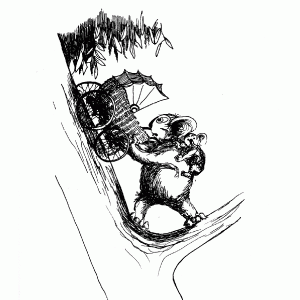By Justin James Zablocki – 2013

Mathematics is the fundamental and abstract study of topics such as quantity, space, change, and structure. Although many people have defined math itself and its scope in somewhat different ways, all mathematicians come to the same final mathematical conclusions. Math rarely changes over time and culture because it is built upon very fundamental and basic tenants and theoretically all true mathematical statements should be able to be proven and false statements disproved in some way. Math has existed in a very basic form longer than recorded history. Historians have found tallies on bones that are prehistoric. More complex math that we can still identify with today can be seen as early as 3000 BC. Since these initial realizations about numbers the field has expanded exponentially. Each concept or part of mathematics that gets added to the field is built upon past tenants and is then later used to create new realizations about math.

You may be asking how exactly math and riddles fit together. Riddles and math are very similar because mathematics is riddles and a lot of riddles are based on mathematical principles. Any math problems can be seen as riddles and the clue to these riddles are the math principles that can be used to solve the problem. Conversely, a lot of riddles can be seen as math problems since they all have to do with linear logic and they often involve numbers themselves. and the same way riddles can be compared to all mathematics because a riddle is solved based on a list of clues and information given to you, just like the method of solving math problems. All mathematical principals and facts are the clues in solving the problem at hand and are used to solve the problem.

In order to see this relationship just look at the equation 1 + 2 does not equal 2 + 3. How do we know the solution to this problem? We know this equation is correct because we know that 1 + 2 = 3, 2 + 3 = 5 and that five and three are not the same number. In this same way all mathematical problems use our previous knowledge to solve for new problems. One great riddle that is also a math problem is the missing dollar riddle. In the riddle three men pay ten dollars each to a hotel for a total of thirty dollars but the hotel realizes they overpaid by five dollars and gives a bellhop the money to repay them. The bellhop pockets two dollars and gives a dollar to each man. Each man paid ten dollars and got one back for a total of twenty-seven dollars paid, then the bellhop kept two dollars for a total of 29 dollars instead of thirty. What happened to the final dollar? By going through the riddle carefully and using mathematical principle it is obvious that there is no missing dollar, the riddle just doesn’t correctly account for all of the money.

Riddles and math go very well together becoming one and the same at some points. They both take logic and intelligence to solve and are a great way to improve your logic and intelligence.

For some math riddles visit Good Riddles Now math section.